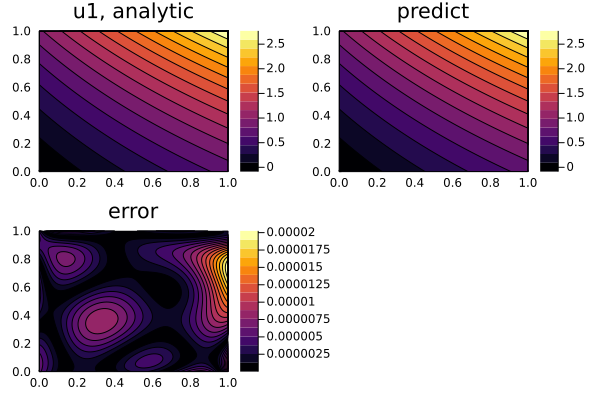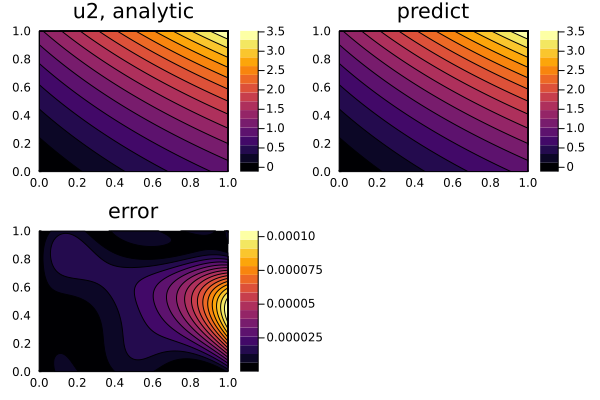# Nonlinear elliptic system of PDEs

We can also solve nonlinear systems such as the system of nonlinear elliptic PDEs

\begin{aligned} \frac{\partial^2u}{\partial x^2} + \frac{\partial^2u}{\partial y^2} = uf(\frac{u}{w}) + \frac{u}{w}h(\frac{u}{w}) \\ \frac{\partial^2w}{\partial x^2} + \frac{\partial^2w}{\partial y^2} = wg(\frac{u}{w}) + h(\frac{u}{w}) \\ \end{aligned}

where f, g, h are arbitrary functions. With initial and boundary conditions:

\begin{aligned} u(0,y) = y + 1 \\ w(1, y) = [cosh(\sqrt[]{f(k)}) + sinh(\sqrt[]{f(k)})]\cdot(y + 1) \\ w(x,0) = cosh(\sqrt[]{f(k)}) + sinh(\sqrt[]{f(k)}) \\ w(0,y) = k(y + 1) \\ u(1, y) = k[cosh(\sqrt[]{f(k)}) + sinh(\sqrt[]{f(k)})]\cdot(y + 1) \\ u(x,0) = k[cosh(\sqrt[]{f(k)}) + sinh(\sqrt[]{f(k)})] \\ \end{aligned}

where k is a root of the algebraic (transcendental) equation f(k) = g(k).

This is done using a derivative neural network approximation.

using NeuralPDE, Lux, ModelingToolkit, Optimization, OptimizationOptimJL, Roots
using Plots
import ModelingToolkit: Interval, infimum, supremum

@parameters x, y
Dx = Differential(x)
Dy = Differential(y)
@variables Dxu(..), Dyu(..), Dxw(..), Dyw(..)
@variables u(..), w(..)

# Arbitrary functions
f(x) = sin(x)
g(x) = cos(x)
h(x) = x
root(x) = f(x) - g(x)

# Analytic solution
k = find_zero(root, (0, 1), Bisection())                            # k is a root of the algebraic (transcendental) equation f(x) = g(x)
θ(x, y) = (cosh(sqrt(f(k)) * x) + sinh(sqrt(f(k)) * x)) * (y + 1)   # Analytical solution to Helmholtz equation
w_analytic(x, y) = θ(x, y) - h(k) / f(k)
u_analytic(x, y) = k * w_analytic(x, y)

# Nonlinear Steady-State Systems of Two Reaction-Diffusion Equations with 3 arbitrary function f, g, h
eqs_ = [Dx(Dxu(x, y)) + Dy(Dyu(x, y)) ~ u(x, y) * f(u(x, y) / w(x, y)) + u(x, y) / w(x, y) * h(u(x, y) / w(x, y)),
Dx(Dxw(x, y)) + Dy(Dyw(x, y)) ~ w(x, y) * g(u(x, y) / w(x, y)) + h(u(x, y) / w(x, y))]

# Boundary conditions
bcs_ = [u(0, y) ~ u_analytic(0, y),
u(1, y) ~ u_analytic(1, y),
u(x, 0) ~ u_analytic(x, 0),
w(0, y) ~ w_analytic(0, y),
w(1, y) ~ w_analytic(1, y),
w(x, 0) ~ w_analytic(x, 0)]

der_ = [Dy(u(x, y)) ~ Dyu(x, y),
Dy(w(x, y)) ~ Dyw(x, y),
Dx(u(x, y)) ~ Dxu(x, y),
Dx(w(x, y)) ~ Dxw(x, y)]

bcs__ = [bcs_;der_]

# Space and time domains
domains = [x ∈ Interval(0.0, 1.0),
y ∈ Interval(0.0, 1.0)]

# Neural network
input_ = length(domains)
n = 15
chain = [Lux.Chain(Dense(input_, n, Lux.σ), Dense(n, n, Lux.σ), Dense(n, 1)) for _ in 1:6] # 1:number of @variables

discretization = PhysicsInformedNN(chain, strategy)

vars = [u(x,y),w(x,y),Dxu(x,y),Dyu(x,y),Dxw(x,y),Dyw(x,y)]
@named pdesystem  = PDESystem(eqs_, bcs__, domains, [x,y], vars)
prob = NeuralPDE.discretize(pdesystem , discretization)
sym_prob = NeuralPDE.symbolic_discretize(pdesystem , discretization)

discretization = PhysicsInformedNN(chain, strategy)
sym_prob = NeuralPDE.symbolic_discretize(pdesystem, discretization)

pde_inner_loss_functions = sym_prob.loss_functions.pde_loss_functions
bcs_inner_loss_functions = sym_prob.loss_functions.bc_loss_functions[1:6]
aprox_derivative_loss_functions = sym_prob.loss_functions.bc_loss_functions[7:end]

callback = function (p, l)
println("loss: ", l)
println("pde_losses: ", map(l_ -> l_(p), pde_inner_loss_functions))
println("bcs_losses: ", map(l_ -> l_(p), bcs_inner_loss_functions))
println("der_losses: ", map(l_ -> l_(p), aprox_derivative_loss_functions))
return false
end

res = Optimization.solve(prob, BFGS(); callback = callback, maxiters=5000)

phi = discretization.phi

# Analysis
xs, ys = [infimum(d.domain):0.01:supremum(d.domain) for d in domains]
depvars = [:u,:w]
minimizers_ = [res.u.depvar[depvars[i]] for i in 1:2]

analytic_sol_func(x,y) = [u_analytic(x, y), w_analytic(x, y)]
u_real  = [[analytic_sol_func(x, y)[i] for x in xs for y in ys] for i in 1:2]
u_predict  = [[phi[i]([x,y], minimizers_[i]) for x in xs for y in ys] for i in 1:2]
diff_u = [abs.(u_real[i] .- u_predict[i]) for i in 1:2]
for i in 1:2
p1 = plot(xs, ys, u_real[i], linetype=:contourf, title="u$i, analytic"); p2 = plot(xs, ys, u_predict[i], linetype=:contourf, title="predict"); p3 = plot(xs, ys, diff_u[i], linetype=:contourf, title="error"); plot(p1, p2, p3) savefig("non_linear_elliptic_sol_u$i")
end